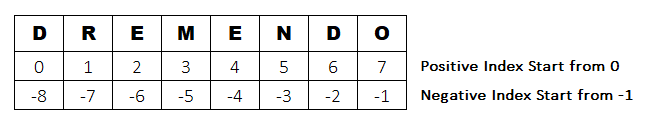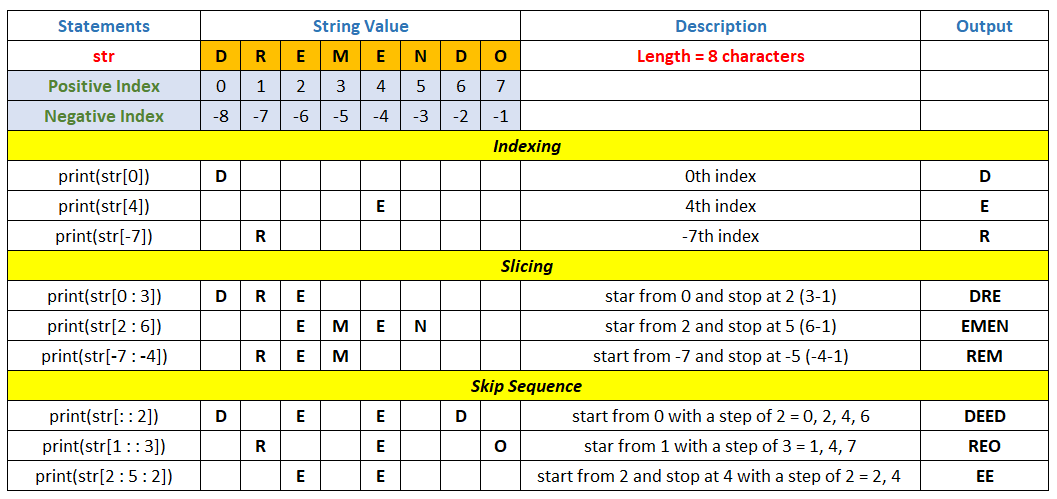# String in Python Programming

###### String in Python

In this lesson, we will understand what is string and its various uses with the help of some examples.

## What is String

String in Python is a sequence of Unicode characters. They are mainly used in Python to store text information. A String is treated as a sequence data type in Python. A sequence data type is a type in which values are stored one after another in computer memory.

Python does not have any character data type. Even a single character is treated as string having a length of one character.

Strings are immutable. It means that we cannot modify the characters of a string directly.

Let’s see how to use the string in several ways in a python program.## Single line String

A single line String can be created in Python either by enclosing it in single or double quotation marks.

#### Example

``````str='DREMENDO'
print(str)
str="Dremendo"
print(str)``````

#### Output

```DREMENDO
Dremendo```

## Multiline String

A multiline String can be created in Python by enclosing it in a three single or double quotation marks.

#### Example

``````str='''We are learning Python
at dremendo.com'''
print(str)
str="""We are learning Python
at dremendo.com"""
print(str)``````

#### Output

```We are learning Python
at dremendo.com
We are learning Python
at dremendo.com```

## Accessing Character in a String

To access any character in a String, we have to use square bracket [] and the index number of the character present in the String. See the image given below to understand the character indexing in a String.We can see here that the positive index of first character starts from 0 and goes from left to right order. Whereas, the negative index of the last character, starts from -1 and goes from right to left order as shown in the above image.

Now to access individual character from the string we have to use the following syntax.

``string_variable[index_number]``

#### Example

``````str='DREMENDO'
print(str)
print(str[-2])``````

```E
D```

## String Slicing

String slicing is the process of accessing a part of the string using either positive or negative indexing with the help of string slicing syntax given below.

``string_variable[ start : end+1 : step]``#### Example

``````str='DREMENDO'
print(str)
print(str)
print(str[-7])
print(str[0:3])
print(str[2:6])
print(str[-7:-4])
print(str[::2])
print(str[1::3])
print(str[2:5:2])``````

```D
E
R
DRE
EMEN
REM
DEED
REO
EE```

## String Concatenation

String Concatenation is the process of joining two or more strings together with the help of + operator.

#### Example

``````str='Python' + ' Programming'
print(str)``````

#### Output

`Python Programming`

## String Repetition

String Repetition creates new strings using * operator and concatenates multiple copies of the same String together.

#### Example

``````str='Dremendo ' * 3
print(str)``````

#### Output

`Dremendo Dremendo Dremendo`

## String Length

The length of a string can be found using the inbuilt function len().

#### Example

``````str='Dremendo'
print('Length =',len(str))``````

#### Output

`Length = 8`

## Iterate all Characters in String using Loop

We can use loop to iterate all characters in a string one by one. Let’s see how.

#### Example 1 (using for loop)

``````str='Dremendo'
for ch in str:
print(ch)``````

#### Output

```D
r
e
m
e
n
d
o```

Here ch is a variable which reads single character from the str variable one by one using for loop and thus printing the output on the screen.

#### Example 2 (print in reverse order using for loop)

``````str='Dremendo'
for x in range(1, len(str)+1):
print(str[x*-1], end='')     # printing the string using negative indexing -1 -2 -3 so on``````

#### Output

`odnemerD`

## Use of Membership Operator in String

Using Membership Operator (in, not in) we can easily check if a substring exists withing a string or not.

#### Example

``````str='Apple'
x='pp' in str       # [pp exists in apple] answer = True (yes)
print(x)
x='px' in str       # [px exists in apple] anwser = False (no)
print(x)
x='mx' not in str   # [mx does not exists in apple] anwser = True (yes)
print(x)``````

```True
False
True```

## Use of Escape Sequences in String

We use escape sequences to insert special character that cannot be written directly inside a string. For example, if we want to print the text like David asked “What’s you name?” then this is possible only using escape sequence or three single quotes. Let’s see how.

#### Example

``````# using single quote escape sequence

# using double quote escape sequence

# using three single quotes

#### Output

```David asked “What's your name?”

## List of Escape Sequence Characters in Python

 Escape Sequence Character Description \n Insert a linefeed (new line) \r Insert a carriage return \f Insert a form feed \b Insert a backspace \t Insert a tab \\ Insert a backslash \" Insert a double quote \' Insert a single quote

## Format String using format() Function

We can format string using string format() function. String formatting is useful when we want to print different type of values inside the string using the placeholder {}.

#### Example

``````a=5
b=12.654
c='Andrew'
d=True
print('We are printing an integer value {} a float value {} a name {} and a Boolean value {}'.format(a,b,c,d))``````

#### Output

`We are printing an integer value 5 a float value 12.654 a name Andrew and a Boolean value True`

So in the above example we can see that the values of a, b, c and d got replaced with the placeholder {} in the same sequence that we have mentioned inside format function.

If we want, we can also use the index number inside placeholders to change the position of the values while printing on the screen.

#### Example

``````a=5
b=12.654
c='Andrew'
d=True
print('We are printing a float value {1}, a boolean value {3}, an integer value {0} and a name {2}'.format(a,b,c,d))``````

#### Output

`We are printing a float value 12.654, a boolean value True, an integer value 5 and a name Andrew`

The first variable a inside the format() function is at index 0, b at index 1, c at index 2 and d at index 3. We have mentioned the index number of the respective variables inside the placeholder {}. That’s why the outputs are printed in the same order.

We can also use name in place of index as shown below.

#### Example

``````a=5
b=12.654
c='Andrew'
d=True
print('We are printing a float value {flt}, a boolean value {boln}, an integer value {intx} and a name {name}'.format(intx=a, flt=b, name=c,boln=d))``````

#### Output

`We are printing a float value 12.654, a boolean value True, an integer value 5 and a name Andrew`

To round up a floating point number up to 2 decimal places we have to use {:.2f} while formatting the output. We can use {:.3f} to round up to 3 decimal places as shown below.

#### Example

``````a=12.364589
print('Round up to 2 decimal places {:.2f}'.format(a))
print('Round up to 3 decimal places {:.3f}'.format(a))``````

#### Output

```Round up to 2 decimal places 12.36
Round up to 3 decimal places 12.365```

## Format String using % Operator

With the help of % operator we can format the values inside the String. This is an old method of formatting a String.

#### Example

``````a=5
b=12.654819
c='Andrew'
d=True
print('Integer=%d  Float=%f  Name=%s  Boolean=%s' %(a,b,c,d))
print('Round up to 2 decimal places %.2f' %(b))
print('Round up to 3 decimal places %.3f' %(b))``````

#### Output

```Integer=5  Float=12.654819  Name=Andrew  Boolean=True
Round up to 2 decimal places 12.65
Round up to 3 decimal places 12.655```

## List of Formatting Symbols

Below are the list of symbols used with % operator for formatting outputs on the screen.

 Formatting Symbols Description %d Used for integer printing %i Used for integer printing %f Used for decimal number (float) printing %c Used for character printing %s Used for string (text) printing %o Used for octal number printing %x Used for lowercase hexadecimal number printing %X Used for uppercase hexadecimal number printing